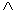# Translations of Encyclopedia about Mathematics

## Boolean Algebra and Logical Circuits

The algebra and logics founded by George Boole has become the foundation of computer technology. The concepts and logical relations of statements are expressed using special symbols in Boolean algebra. The laws of algebra can also be applied to .

While the truth values of TRUE or FALSE are decisive with assertion or predicative logics, in algebra of logical circuits, the values of 0 and 1 fulfil this role. The relation of sets also apply here as applies with assertion logic functions.

and – conjunction         is expressed using “Ù” or “&

OR – disjunction            is expressed using “Ú” or “+”

NO – negation               is expressed using “-“ or “Ø” or “~” before the quantity

Boolean operators refer to the set of all subsets of a particular set. Some of the logical connections between sets are Ç - which refers to the intersection of sets or for the function and; È – which is used to unify sets or the function OR.

In logics, computer circuits use the binary values of 0 and 1 instead of THE TRUE and FALSE of assertion logics. For example, 1 may describe the statement “current is turned on” and 0 “current is turned off”. According to the type of connection, the combination of A and B can generate a result of either 0 or 1.

Conjunction

Also referred to as the function and (or yes-switch).

Q = A